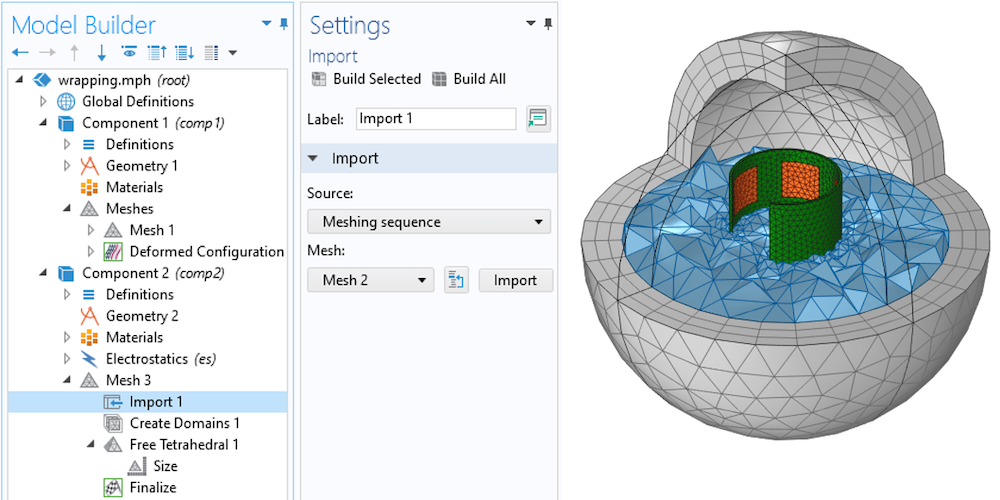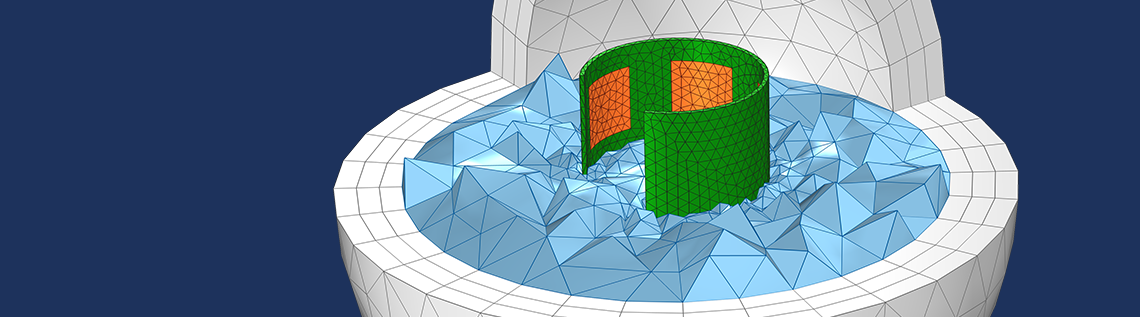# 如何对卷绕和翘曲几何形状进行建模？

2021年 9月 10日

### 定义变形形状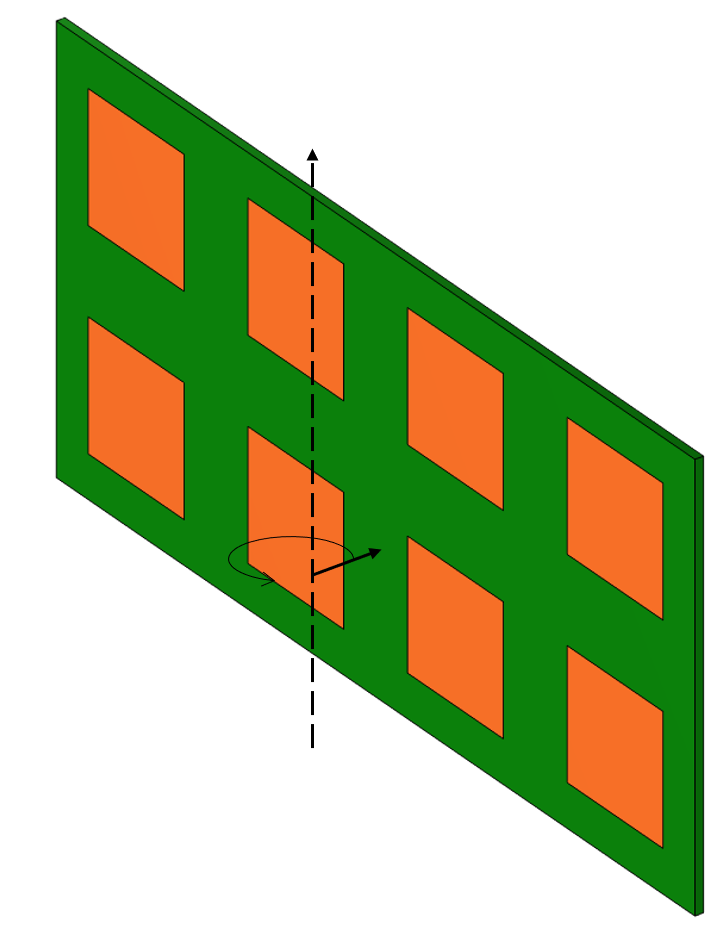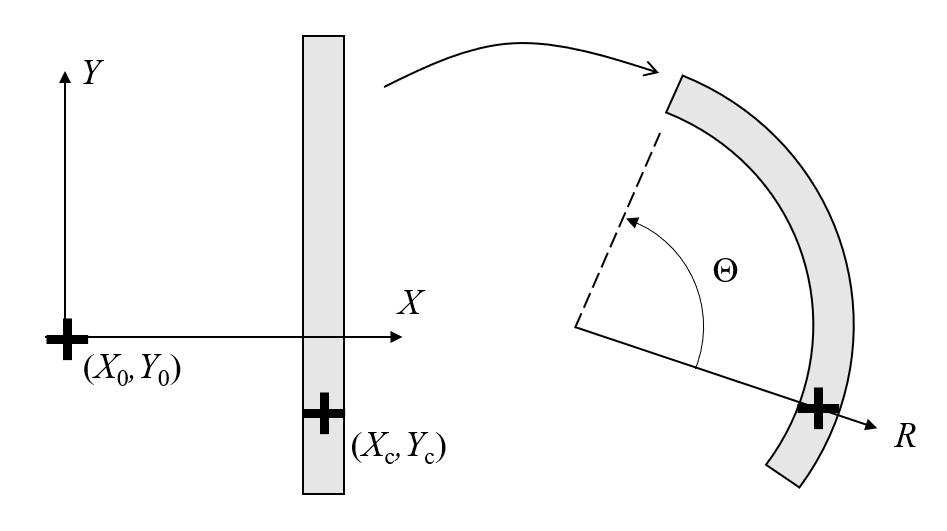R=(X-X_c)+R_0
\Theta = (Y-Y_c)/R_0 + \text{atan2}(Y_c-Y_0,X_c-X_0)

dx = R \cos(\Theta) – (X-X_0)
dy = R \sin(\Theta) – (Y-Y_0)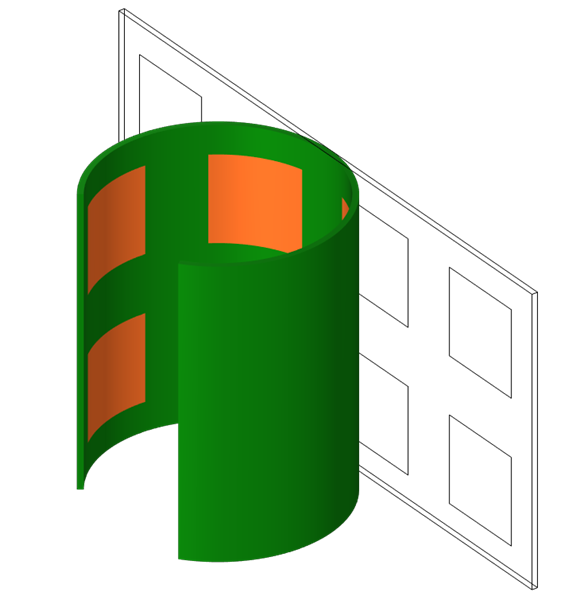### 通过第二个组件和网格对周围域进行网格划分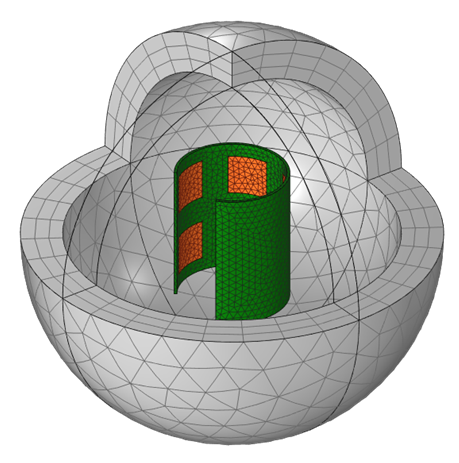1. 卷绕的部分现在有一个变形的网格
2. 紧邻的周围空间没有网格